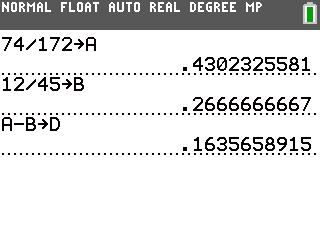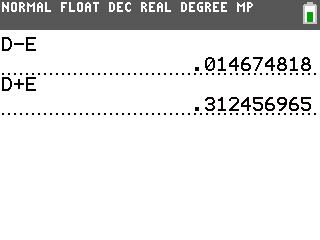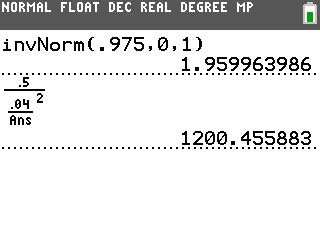••• ##### Device
• TI-83 Plus Family
• TI-84 Plus
• TI-84 Plus Silver Edition
•TI-84 Plus C Silver Edition
•TI-84 Plus CE
• ##### Report an Issue

Statistics: Difference Between Two Proportions
by Texas Instruments#### Overview

Students use confidence intervals to estimate the difference of two population proportions.

#### Key Steps

•Problem 1, students estimate the true difference between two population proportions in order to introduce the formulas for the margin of error and confidence interval.

The scenario describes the numbers of men and women that used coupons at a grocery store. Students use the TI-84 Plus C to find each sample proportion and the difference between the sample proportions.

•Students construct the confidence interval by subtracting from and adding to the difference of the sample proportions.

•Students can then find the margin of error. They will first need to use the invNorm command to calculate the z score.

Students should store all their values for future calculations.